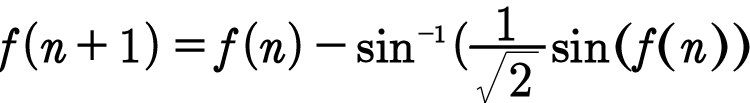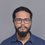# Well, this baffles me!The most scary things that I find in mathematics are functional equations, as there is no general method for solving them as far as I know. The purpose of this note is to gather some information about how to solve these type of equations, and most importantly, whether every functional equation has a solution or not. I know that some of my fellow brilliant members know about this, so help me! As for an example, I encountered this one when I was working on something confidential.Note by Satyam Bhardwaj
6 years, 8 months ago

This discussion board is a place to discuss our Daily Challenges and the math and science related to those challenges. Explanations are more than just a solution — they should explain the steps and thinking strategies that you used to obtain the solution. Comments should further the discussion of math and science.

When posting on Brilliant:

• Use the emojis to react to an explanation, whether you're congratulating a job well done , or just really confused .
• Ask specific questions about the challenge or the steps in somebody's explanation. Well-posed questions can add a lot to the discussion, but posting "I don't understand!" doesn't help anyone.
• Try to contribute something new to the discussion, whether it is an extension, generalization or other idea related to the challenge.
• Stay on topic — we're all here to learn more about math and science, not to hear about your favorite get-rich-quick scheme or current world events.

MarkdownAppears as
*italics* or _italics_ italics
**bold** or __bold__ bold
- bulleted- list
• bulleted
• list
1. numbered2. list
1. numbered
2. list
Note: you must add a full line of space before and after lists for them to show up correctly
paragraph 1paragraph 2

paragraph 1

paragraph 2

[example link](https://brilliant.org)example link
> This is a quote
This is a quote
    # I indented these lines
# 4 spaces, and now they show
# up as a code block.

print "hello world"
# I indented these lines
# 4 spaces, and now they show
# up as a code block.

print "hello world"
MathAppears as
Remember to wrap math in $$ ... $$ or $ ... $ to ensure proper formatting.
2 \times 3 $2 \times 3$
2^{34} $2^{34}$
a_{i-1} $a_{i-1}$
\frac{2}{3} $\frac{2}{3}$
\sqrt{2} $\sqrt{2}$
\sum_{i=1}^3 $\sum_{i=1}^3$
\sin \theta $\sin \theta$
\boxed{123} $\boxed{123}$

## Comments

Sort by:

Top Newest

I can't solve it either. I suppose you don't know f(0) or f(1)? I find that the behaviour of this equation depends strongly on the boundary value. If the first value is 0 then all subsequent values are (as you have doubtless noticed). If the first is just $\pi$ then subsequent terms are $\pi$. If the first term is a fairly small fraction of $\pi$ then subsequent terms drop off to zero quickly. If the first term is 1.25 times $\pi$ then subsequent terms rise quickly to twice $\pi$. Incidentally I used this code to do my little investigation.- 6 years, 4 months ago

Log in to reply

Only if i knew programming! Anyways, as I have written in the post, im not going to tell what this equation is about, but it would be great help if you could do some analysis on it.... I'll give u credit i find a major breakthrough! (Although thats highly unlikely!)

- 6 years, 4 months ago

Log in to reply

Did you graph it???

- 6 years, 4 months ago

Log in to reply

Yes, that's right. You can explore this recurrence without programming using http://www.wolframalpha.com/. I just tried this by entering

plot f(n+1)=f(n)-arcsin(sin(f(n))/sqrt(2)),f(1)=pi/4

You can see the initial condition at the right.

I'll have to admit at the outset that my analysis skills are limited. I like numerical methods; I'm not at all skilled or knowledgeable in algebra.

- 6 years, 4 months ago

Log in to reply

My actual aim with this problem is to find the general term of this sequence. I can plot the graph to see how it varies but it will not help me solve it unless i superimpose it with thousands of graphs to see which fits! I can tell you what this is about but not on brilliant....

- 6 years, 4 months ago

Log in to reply

Have you considered asking for a solution on quora.com? There are people on there orders of magnitude more able than I am.

- 6 years, 4 months ago

Log in to reply

Thanks.. will surely try!

- 6 years, 4 months ago

Log in to reply

×

Problem Loading...

Note Loading...

Set Loading...